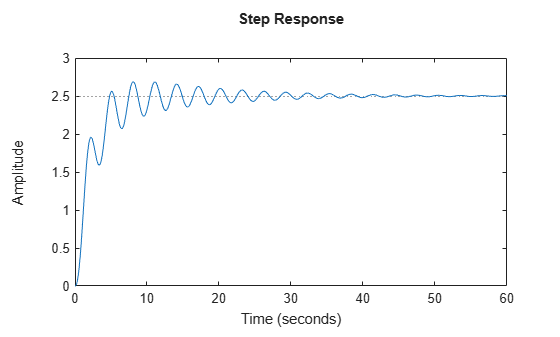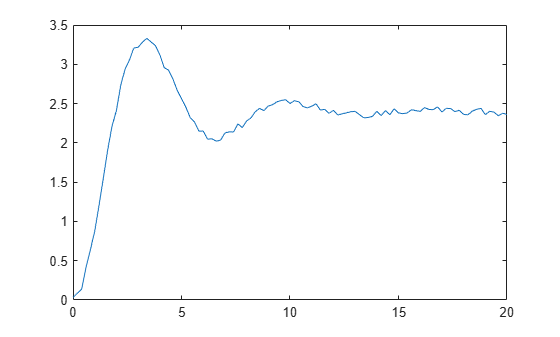# stepinfo

Rise time, settling time, and other step-response characteristics

## Syntax

``S = stepinfo(sys)``
``S = stepinfo(y,t)``
``S = stepinfo(y,t,yfinal)``
``S = stepinfo(___,'SettlingTimeThreshold',ST)``
``S = stepinfo(___,'RiseTimeLimits',RT)``

## Description

example

````S = stepinfo(sys)`computes the step-response characteristics for a dynamic system model `sys`. The function returns the characteristics in a structure containing the fields:`RiseTime` — Time it takes for the response to rise from 10% to 90% of the steady-state response.`SettlingTime` — Time it takes for the error |y(t) - yfinal| between the response y(t) and the steady-state response yfinal to fall to within 2% of yfinal.`SettlingMin` — Minimum value of y(t) once the response has risen.`SettlingMax` — Maximum value of y(t) once the response has risen.`Overshoot` — Percentage overshoot, relative to yfinal.`Undershoot` — Percentage undershoot.`Peak` — Peak absolute value of y(t)`PeakTime` — Time at which the peak value occurs.The following figure illustrates some of these quantities on a typical second-order response.Using this syntax requires a Control System Toolbox™ license.```
````S = stepinfo(y,t)` computes step-response characteristics from an array of step-response data `y` and corresponding time vector `t`. For SISO system responses, `y` is a vector with the same number of entries as `t`. For MIMO response data, `y` is an array containing the responses of each I/O channel. This syntax uses the last value in `y` (or the last value in each channel's corresponding response data) as the steady-state value for computing characteristics that depend on that value.```

example

````S = stepinfo(y,t,yfinal)` computes step-response characteristics relative to the steady-state value `yfinal`. This syntax is useful when you know that the expected steady-state system response differs from the last value in `y` for reasons such as measurement noise.For SISO responses, `t` and `y` are vectors with the same length NS. For systems with NU inputs and NY outputs, you can specify `y` as an NS-by-NY-by-NU array (see `step`) and `yfinal` as an NY-by-NU array. `stepinfo` then returns a NY-by-NU structure array `S` of performance metrics for each I/O pair.```

example

````S = stepinfo(___,'SettlingTimeThreshold',ST)` lets you specify the threshold `ST` used in the definition of settling time. The response has settled when the error ```|y(t) - yfinal|``` becomes smaller than a fraction `ST` of its peak value. The default value is `ST = 0.02` (2%). You can use this syntax with any of the previous input-argument combinations. ```

example

````S = stepinfo(___,'RiseTimeLimits',RT)` lets you specify the lower and upper thresholds used in the definition of rise time. By default, the rise time is defined as the time the response takes to rise from 10 to 90% of the steady-state value (```RT = [0.1 0.9]```). The upper threshold `RT(2)` is also used to calculate `SettlingMin` and `SettlingMax`. These values are the minimum and maximum values of the response occurring after the response has reached the upper threshold. You can use this syntax with any of the previous input-argument combinations. ```

## Examples

collapse all

Compute step-response characteristics such as rise time, settling time, and overshoot for a dynamic system model. For this example, use the continuous-time transfer function:

`$sys=\frac{{s}^{2}+5s+5}{{s}^{4}+1.65{s}^{3}+5{s}^{2}+6.5s+2}.$`

Create the transfer function and examine its step response.

```sys = tf([1 5 5],[1 1.65 5 6.5 2]); step(sys)```The plot shows that the response rises in a few seconds, and then rings down to a steady-state value of about 2.5. Compute the characteristics of this response using `stepinfo`.

`S = stepinfo(sys)`
```S = struct with fields: RiseTime: 3.8456 SettlingTime: 27.9762 SettlingMin: 2.0689 SettlingMax: 2.6873 Overshoot: 7.4915 Undershoot: 0 Peak: 2.6873 PeakTime: 8.0530 ```

By default, the settling time is the time it takes for $y\left(t\right)-{y}_{final}$ to fall below 2% of its peak value, where $y\left(t\right)$is the system response at time t and ${y}_{final}$ is the steady-state response. The result `S.SettlingTime` shows that for `sys`, this condition occurs after about 28 seconds. The default definition of rise time is the time it takes for the response to go from 10% of its steady-state value to 90% of that value. `S.RiseTime` shows that for `sys`, this rise occurs in less than 4 seconds. The maximum overshoot is returned in `S.Overshoot`. For this system, the peak value `S.Peak`, which occurs at the time `S.PeakTime`, overshoots the steady-state value by about 7.5% of the steady-state value.

For a MIMO system, `stepinfo` returns a structure array in which each entry contains the response characteristics of the corresponding I/O channel of the system. For this example, use a two-output, two-input discrete-time system. Compute the step-response characteristics.

```A = [0.68 -0.34; 0.34 0.68]; B = [0.18 -0.05; 0.04 0.11]; C = [0 -1.53; -1.12 -1.10]; D = [0 0; 0.06 -0.37]; sys = ss(A,B,C,D,0.2); S = stepinfo(sys)```
```S=2×2 struct array with fields: RiseTime SettlingTime SettlingMin SettlingMax Overshoot Undershoot Peak PeakTime ```

Access the response characteristics for a particular I/0 channel by indexing into `S`. For instance, examine the response characteristics for the response from the first input to the second output of `sys`, corresponding to `S(2,1)`.

`S(2,1)`
```ans = struct with fields: RiseTime: 0.4000 SettlingTime: 2.8000 SettlingMin: -0.6724 SettlingMax: -0.5188 Overshoot: 24.6476 Undershoot: 11.1224 Peak: 0.6724 PeakTime: 1 ```

To access a particular value, use dot notation. For instance, extract the rise time of the (2,1) channel.

`rt21 = S(2,1).RiseTime`
```rt21 = 0.4000 ```

By default, `stepinfo `defines settling time as the time it takes for the error $|\mathit{y}\left(\mathit{t}\right)-{\mathit{y}}_{\mathit{final}}|$ between the response $\mathit{y}\left(\mathit{t}\right)$and the steady-state response ${\mathit{y}}_{\mathit{final}}$ to come within 2% of ${\mathit{y}}_{\mathit{final}}$. Also, `stepinfo` defines the rise time as the time it takes for the response to rise from 10% of ${\mathit{y}}_{\mathit{final}}$ to 90% of ${\mathit{y}}_{\mathit{final}}$. You can change these definitions using `SettlingTimeThreshold` and `RiseTimeThreshold`. For this example, use the system given by:

`$\mathit{sys}=\frac{{\mathit{s}}^{2}+5\mathit{s}+5}{{\mathit{s}}^{4}+1.65{\mathit{s}}^{3}+6.5\mathit{s}+2}.\text{\hspace{0.17em}}$`

Create the transfer function.

`sys = tf([1 5 5],[1 1.65 5 6.5 2]);`

Compute the time it takes for the error in the response of `sys` to to reach 0.5% of the steady-state response. To do so, set `SettlingTimeThreshold` to 0.5%, or 0.005.

```S1 = stepinfo(sys,'SettlingTimeThreshold',0.005); st1 = S1.SettlingTime```
```st1 = 46.1325 ```

Compute the time it takes the response of `sys `to rise from 5% to 95% of the steady-state value. To do so, set `RiseTimeThreshold` to a vector containing those bounds.

```S2 = stepinfo(sys,'RiseTimeThreshold',[0.05 0.95]); rt2 = S2.RiseTime```
```rt2 = 4.1690 ```

You can define both settling time and rise time in the same computation.

`S3 = stepinfo(sys,'SettlingTimeThreshold',0.005,'RiseTimeThreshold',[0.05 0.95])`
```S3 = struct with fields: RiseTime: 4.1690 SettlingTime: 46.1325 SettlingMin: 2.0689 SettlingMax: 2.6873 Overshoot: 7.4915 Undershoot: 0 Peak: 2.6873 PeakTime: 8.0530 ```

You can extract step-response characteristics from step-response data even if you do not have a model of your system. For instance, suppose you have measured the response of your system to a step input, and saved the resulting response data in a vector `y` of response values at the times stored in another vector, `t`. Load the response data and examine it.

```load StepInfoData t y plot(t,y)```Compute step-response characteristics from this response data using `stepinfo`. If you do not specify the steady-state response value `yfinal`, then `stepinfo` assumes that the last value in the response vector `y` is the steady-state response`.`Because there is some noise in the data, the last value in `y` is likely not the true steady-state response value. When you know what the steady-state value should be, you can provide it to `stepinfo`. For this example, suppose that the steady-state response is 2.4.

`S1 = stepinfo(y,t,2.4)`
```S1 = struct with fields: RiseTime: 1.2713 SettlingTime: 19.6478 SettlingMin: 2.0219 SettlingMax: 3.3302 Overshoot: 38.7575 Undershoot: 0 Peak: 3.3302 PeakTime: 3.4000 ```

Because of the noise in the data, the default definition of the settling time is too stringent, resulting in an arbitrary value of almost 20 seconds. To allow for the noise, increase the settling-time threshold from the default 2% to 5%.

`S2 = stepinfo(y,t,2.4,'SettlingTimeThreshold',0.05)`
```S2 = struct with fields: RiseTime: 1.2713 SettlingTime: 10.4201 SettlingMin: 2.0219 SettlingMax: 3.3302 Overshoot: 38.7575 Undershoot: 0 Peak: 3.3302 PeakTime: 3.4000 ```

## Input Arguments

collapse all

Dynamic system, specified as a SISO or MIMO dynamic system model. Dynamic systems that you can use include:

• Continuous-time or discrete-time numeric LTI models, such as `tf`, `zpk`, or `ss` models.

• Generalized or uncertain LTI models such as `genss` or `uss` (Robust Control Toolbox) models. (Using uncertain models requires Robust Control Toolbox™ software.) For generalized models, `stepinfo` computes the step-response characteristics using the current value of tunable blocks and the nominal value of uncertain blocks.

• Identified LTI models, such as `idtf` (System Identification Toolbox), `idss` (System Identification Toolbox), or `idproc` (System Identification Toolbox) models. (Using identified models requires System Identification Toolbox™ software.)

Step-response data, specified as:

• For SISO response data, a vector of length `Ns`, where `Ns` is the number of samples in the response data.

• For MIMO response data, an `Ns`-by-`Ny`-by-`Nu` array, where `Ny` is the number of system outputs, and `Nu` is the number of system inputs.

Time vector corresponding to the response data in `y`, specified as a vector of length `Ns`.

• For SISO response data, a scalar value.

• For MIMO response data, an `Ny`-by-`Nu` array, where each entry provides the steady-state response value for the corresponding system channel.

If you do not provide `yfinal`, then `stepinfo` uses the last value in the corresponding channel of `y` as the steady-state response value.

Threshold for defining settling time, specified as a scalar value between 0 and 1. By default, `stepinfo` defines settling time as the time it takes for the error |y(t) - yfinal| between the response y(t) and the steady-state response yfinal to fall to within 2% of yfinal. To change this definition, set `ST` to a different value. For instance, to set a threshold of 5%, set `ST` to 0.05.

Threshold for defining rise time, specified as a 2-element row vector of nondescending values between 0 and 1. By default, `stepinfo` defines rise time as the time it takes for the response to rise from 10% to 90% of the steady-state value yfinal. To change this definition, set `RT` to a different value. For instance, to define the rise time as the time it takes for the response to rise from 5% to 95% of the steady-state value, set `RT` to `[0.05 0.95]`.

## Output Arguments

collapse all

Step-response characteristics of `sys`, returned as a structure containing the fields:

• `RiseTime` — Time it takes for the response to rise from 10% to 90% of the steady-state response.

• `SettlingTime` — Time it takes for the error |y(t) - yfinal| between the response y(t) and the steady-state response yfinal to fall to within 2% of yfinal.

• `SettlingMin` — Minimum value of y(t) once the response has risen.

• `SettlingMax` — Maximum value of y(t) once the response has risen.

• `Overshoot` — Percentage overshoot, relative to yfinal).

• `Undershoot` — Percentage undershoot.

• `Peak` — Peak absolute value of y(t)

• `PeakTime` — Time at which the peak value occurs.

For MIMO models or responses data, `S` is a structure array in which each entry contains the step-response characteristics of the corresponding I/O channel. For instance, if you provide a 3-input, 3-output model or array of response data, then `S(2,3)` contains the characteristics of the response from the third input to the second output. For an example, see Step-Response Characteristics of MIMO System.

If `sys` is unstable, then all step-response characteristics are `NaN`, except for `Peak` and `PeakTime`, which are `Inf`.